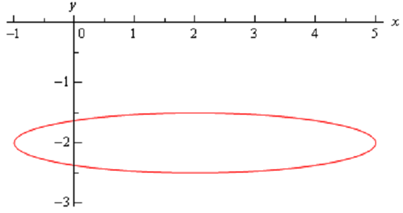Draw a common graph ( x - 2)2 /9+4(y + 2)2 =1, Mathematics

Assignment Help:

Graph     ( x - 2)2 /9+4(y + 2)2  = 1

Solution

It is an ellipse. The standard form of the ellipse is

( x - h )2 /a2 + (y - k )2 / b2 = 1

It is an ellipse with center (h, k) and the right most & left most points are distance of away from the center and the top most & bottom most of the points are a distance of b away from the center.

The ellipse for this equation has center (2, -2) and has a = 3 & b = 1 .  Note  as well that to get the b .we're actually rewriting the equation as,

( x - 2 )2 /9 + (y + 2 )2 / (1/4) = 1

to get it in standard from.

Following is a sketch of the ellipse.Demand Forecast, How should shoppers Stop develop its demand forecasts?

How should shoppers Stop develop its demand forecasts?

p=0

Regression, regression line drawn as Y=C+1075x, when x was 2, and y was 239...

regression line drawn as Y=C+1075x, when x was 2, and y was 239, given that y intercept was 11. calculate the residual

Polynomials, zeroes of polynomial 2x2-3x-2

zeroes of polynomial 2x2-3x-2

One of these food groups, In a collection of 30 dissimilar birds, 15 eat wo...

In a collection of 30 dissimilar birds, 15 eat worms, 18 eat fruit, and 12 eat seeds. Accurately 8 eat worms and seeds, 8 eat worms and fruit, 7 eat fruit and seeds, and 4 eat each

Management, An investment manager at TD Ameritrade is making a decision abo...

An investment manager at TD Ameritrade is making a decision about a \$10,000,000 investment. There are four portfolio options available and she is looking at annual return of these

table of 12

Triangles, if triangle abc is similar to def and ab/de=3/4 find the ratio a...

if triangle abc is similar to def and ab/de=3/4 find the ratio af their perimeter and area

If a sequence is bounded and monotonic then it is convergent, Theorem ...

Theorem If {a n } is bounded and monotonic then { a n } is convergent.  Be cautious to not misuse this theorem.  It does not state that if a sequence is not bounded and/or

Second order differential equations, In the earlier section we looked at fi...

In the earlier section we looked at first order differential equations. In this section we will move on to second order differential equations. Just as we did in the previous secti